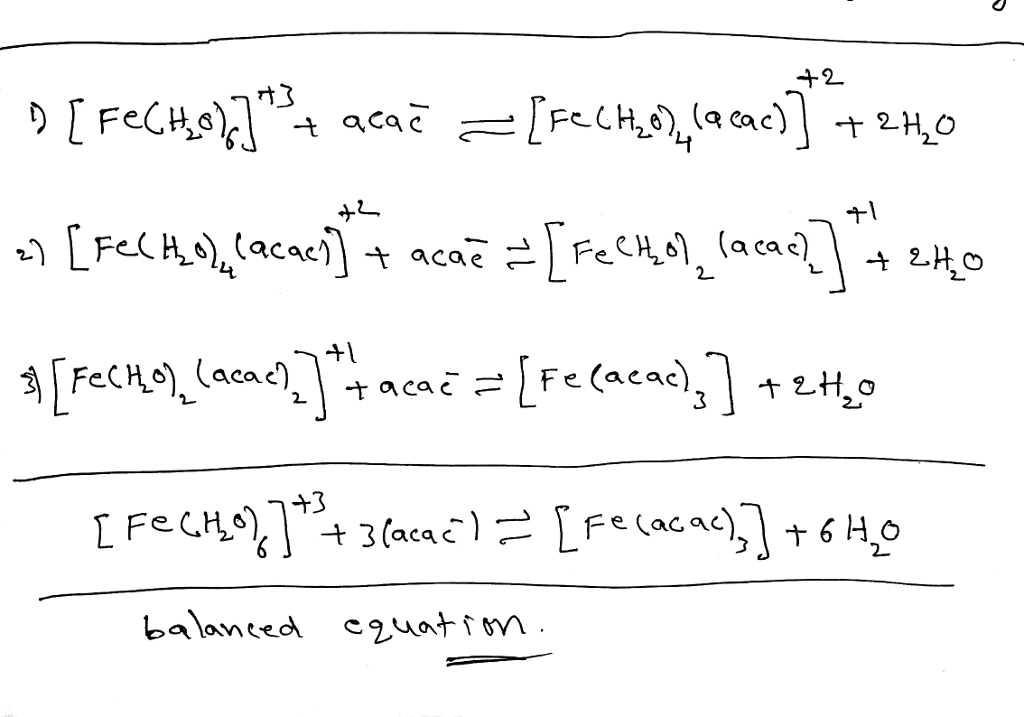# Homework Solution: Complex the equations that describe the stepwise formation of the tris-acac iron…

Fill in the blank. Also can you show me the balanced equation also...? Thx in advance!
Complex the equations that describe the stepwise formation of the tris-acac iron complex, [Fe(acac)_3]: [Fe(H_2O)_6]^3+ + acac^- [Fe(H_2O)_4(acac)]^2+ + 2H_2O [Fe(acac)_3] + 2H_2O